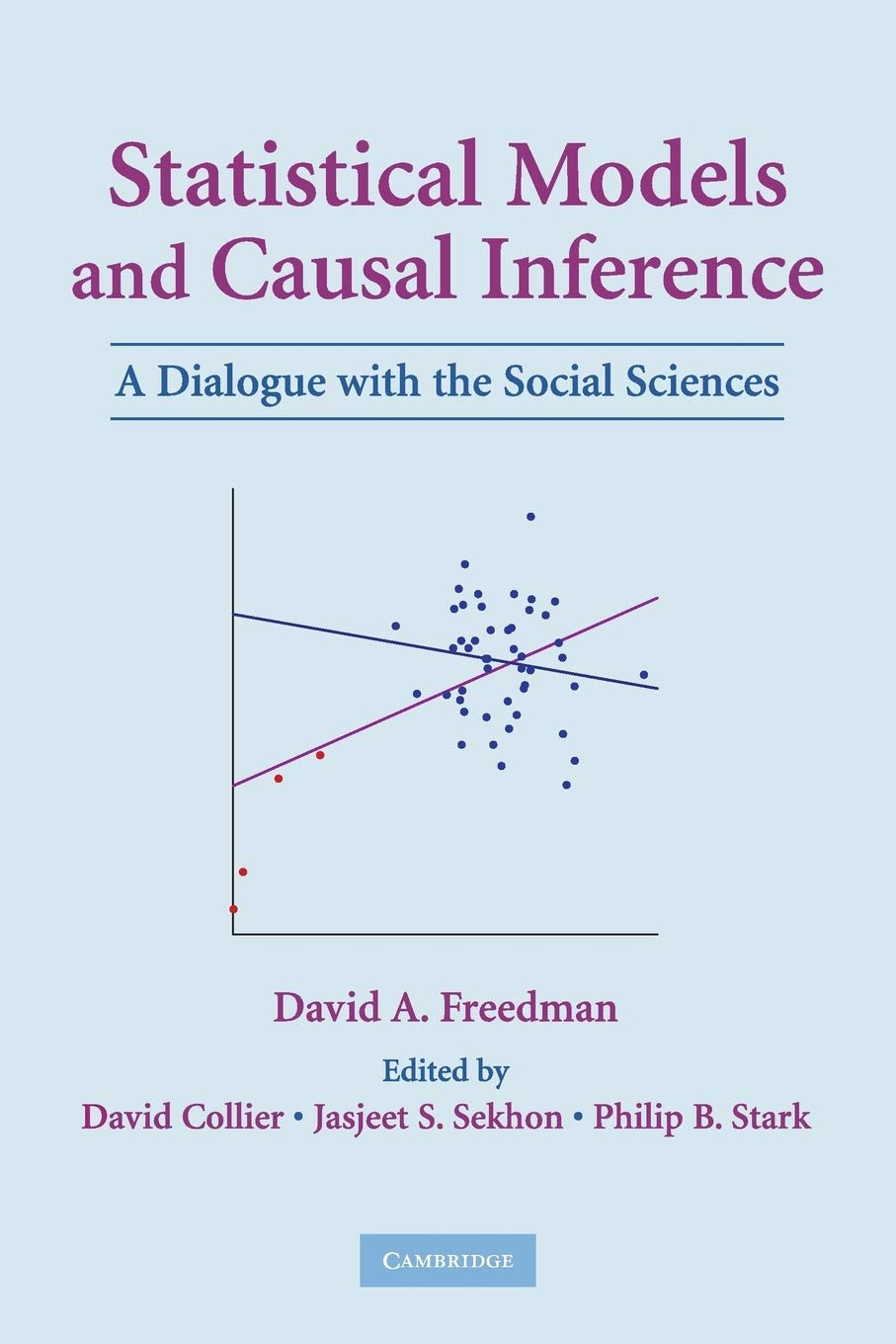## When do regressions give us causality?The issue boils down to this. Does the conditional distribution of Y given X represent mere association, or does it represent the distribution Y would have had if we had intervened and set the values of X? There is a similar question for the distribution of Z given X and Y. These questions cannot be answered just by fitting the equations and doing data analysis on X, Y, and Z. Additional information is needed. From this perspective, the equations are “structural” if the conditional distributions inferred from the equations tell us the likely impact of interventions, thereby allowing a causal rather than an associational interpretation. The take-home message will be clear: You cannot infer a causal relationship from a data set by running regressions—unless there is substantial prior knowledge about the mechanisms that generated the data …